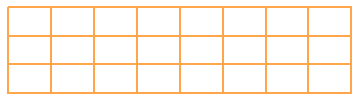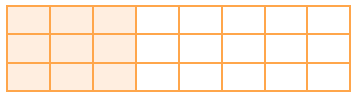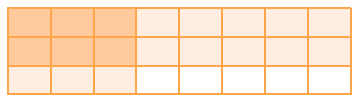### Home > CC2MN > Chapter 3 > Lesson 3.2.3 > Problem3-62

3-62.

Simplify the following fraction expressions. Show all of your work.

1. $\frac{5}{6}+\frac{1}{4}$

Draw diagrams to represent each fraction.Now change the diagram so that each whole is divided into equal portions.

1. $\frac{7}{12}-\frac{2}{5}$

Follow the steps in part (a) to solve the problem.

1. $\frac{3}{8}\cdot\frac{2}{3}$

Draw a rectangle. A rectangle divided into 24 equal sections arranged in 3 rows and 8 columns.Shade in three eighths.  The same rectangle with three sections shaded in each row.

Shade in two thirds in the other direction. The same rectangle with 2 rows lightly shaded, and 6 sections, in those 2 rows, are shaded dark.

1. $\frac{4}{5}\cdot\frac{7}{8}$
$\frac{28}{40}$